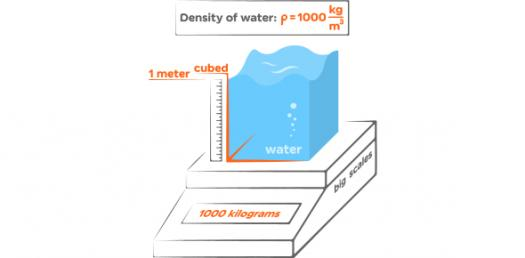# Describing And Measuring Matter! Ultimate Trivia Quiz

17 Questions | Attempts: 207SettingsBelow us the ultimate trivia quiz on describing and measuring matter. If you are a chemistry student you must know that it is indeed possible to measure matter. Matter is essentially anything that has mass and occupies space and this means it can undergo different changes too. Take up this quiz and see if you can which changes are described.

• 1.
A ________ property is a characteristic of a pure substance that can be observed without changing it into another substance.
• A.

Homogeneous

• B.

Heterogeneous

• C.

Chemical

• D.

Physical

• 2.
Table salt is an example of a ____________________.
• A.

Heterogeneous mixture

• B.

Solution

• C.

Compound

• D.

Element

• 3.
Captain Crunch cereal with crunch-berries is an example of a __________________.
• A.

Heterogenous mixture

• B.

Solution

• C.

Element

• D.

Compound

• 4.
The air in the classroom right now is an example of _____________________.
• A.

Heterogenous mixture

• B.

Solution

• C.

Element

• D.

Compound

• 5.
Black coffee is an example of a ______________________.
• A.

Heterogeneous mixture

• B.

Solution

• C.

Element

• D.

Compound

• 6.
Rusting is an example of a _________________.
• A.

Physical property

• B.

Chemical property

• C.

Substance

• D.

Compound

• 7.
Water freezing from liquid to ice is an example of a ________________________.
• A.

Physical property

• B.

Chemical property

• C.

Solution

• D.

Compound

• 8.
New substances are always formed when matter undergoes a ____________________.
• A.

Change in shape

• B.

Physical change

• C.

Change in temperature

• D.

Chemical change

• 9.
Atoms that combine to form larger particles are called ____________________.
• A.

Elements

• B.

Substances

• C.

Molecules

• D.

Chemical bonds

• 10.
What is the ratio of Hydrogen (H) to Oxygen molecules in the following chemical formula H3O
• A.

1:3

• B.

3:1

• C.

3:0

• D.

0:3

• 11.
The measurement of the force of gravity on an object is the object’s
• A.

Mass

• B.

Volume

• C.

Weight

• D.

Density

• 12.
The measurement of how much matter an object contains is its
• A.

Volume

• B.

Weight

• C.

Mass

• D.

Melting point

• 13.
The measurement of how much mass is contained in a given volume is called
• A.

Mass

• B.

Volume

• C.

Weight

• D.

Density

• 14.
The density of a block of wood with a volume of 50 cubic centimeters and a mass of 100 grams is
• A.

2 g/cm3

• B.

0.5 g/cm3

• C.

500 g/cm3

• D.

5,000 g/cm3

• 15.
The volume of an irregular object can be measured by
• A.

Multiplying the object’s length, width, and height.

• B.

Dividing the object’s density by its mass.

• C.

Submerging the object in water in a graduated cylinder.

• D.

Placing the object on a triple-beam balance.

• 16.
Anything that has mass and takes up space is called
• A.

Matter

• B.

Density

• C.

Heterogeneous.

• D.

Homogeneous.

• 17.
How would you calculate the density of an object?
• A.

Divide its weight by its volume.

• B.

Divide its mass by its volume.

• C.

Multiply its volume times its mass.

• D.

Multiply its weight times its mass.

## Related TopicsBack to top
×

Wait!
Here's an interesting quiz for you.